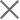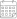Subscribe

### Indexes

Quarter 1-2, 2021 | Energy Market Review24 January 2022

In the first and the second quarters of 2021, Georgian power plants generated 2,429 mln. and 2,708 mln. kWh of electricity, respectively (Figure 1). This represents a 16.0% and 3.2% decrease in total generation compared to the corresponding periods of the previous year (in 2020, the total generation in Q1 was 2,893 mln. kWh and in Q2 it was 2,797 mln. kWh). On an annual basis, the reduction in generation in the first quarter of 2021 derived from an 18.3%, 13.8%, and 16.0% decline in thermal, hydro, and wind power generation, respectively. The decrease in production in the second quarter is related to the 22.8%, 0.7%, and 2.6% decrease in thermal, hydro, and wind power generation, respectively.

In the first and second quarters of 2021, electricity consumption on the local market amounted to 3,469 mln. (Q1) and 3,331 mln. kWh (Q2) (-0.6% and +15.5% compared to the first and second quarters of 2020, respectively) (Figure 1). Total power generation lagged the consumption by 1,040 mln. kWh in the first quarter of the year and by 623 mln. kWh in the second quarter. In percentage terms, in Q1 there was a generation deficit amounting to 42.8% of total generation, while in Q2 there was a generation deficit of 23.0% of total generation. In 2020 the difference between total generation and consumption resulted in a shortage in the first quarter of 598 mln. kWh, around 20.7% of the total generation, and in the second quarter shortage of 86 mln. kWh, around 3.1% of the total generation.